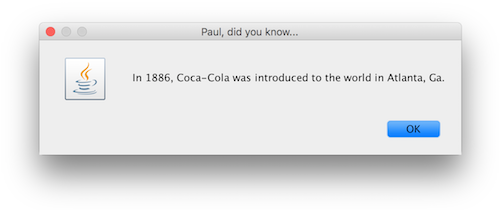## Exercises - Scanner, Random, and File Classes

Some of the exercises below provide sample runs to better clarify what the program in question is supposed to do. In these sample runs, text given in green has been typed by the user, while white text has been output by the program. Additionally, the "$" symbol indicates the command prompt, while the "↵" symbol indicates the user has pressed the return key. 1.  import java.util.Scanner; public class Greeter { public static void main(String[] args) { Scanner scanner = new Scanner(System.in); System.out.println("Enter your name:"); String name = scanner.next(); scanner.close(); // we are done collecting input System.out.println("Hello " + name + "!"); } }  2.  import java.util.Random; import java.util.Scanner; public class RandomPoint { public static void main(String[] args) { // used to generate random numbers.. Random random = new Random(); // used to collect input from user.. Scanner scanner = new Scanner(System.in); System.out.println("minimum x ? "); double minX = scanner.nextDouble(); System.out.println("maximum x ? "); double maxX = scanner.nextDouble(); System.out.println("minimum y ? "); double minY = scanner.nextDouble(); System.out.println("maximum y ? "); double maxY = scanner.nextDouble(); // we are done collecting input.. scanner.close(); double x = minX + random.nextDouble() * (maxX - minX); double y = minY + random.nextDouble() * (maxY - minY); System.out.println("(" + x + ", " + y + ")"); } }  3. Write a class named UnitVector that produces a unit-length vector from a randomly generated point with$x$and$y$coordinates between$0$and$500$to a user-specified point in the same region of the plane, in a manner consistent with the example below. Note: the coordinates of your final vector should be approximated to two decimal places. $ java UnitVector↵
This program will give a unit-length vector
that points from (315,102) to the point
with integer coordinates (with x and y
between 0 and 500 each) that you specify

x? 250
y? 250

unit-length vector towards your point = (-0.40,0.92)

4. 
import java.util.Random;
import java.util.Scanner;

public class StrangeDice {

public static void main(String[] args) {

// used to generate random numbers..
Random random = new Random();

// used to collect input from user..
Scanner scanner = new Scanner(System.in);

System.out.println("Enter some number of sides for the dice: ");
int numSides = scanner.nextInt();

// we are done collecting input..
scanner.close();

int firstDieValue = random.nextInt(numSides) + 1;
int secondDieValue = random.nextInt(numSides) + 1;

int diceTotal = firstDieValue + secondDieValue;

System.out.println("You rolled a " + diceTotal);
System.out.println("(" + firstDieValue + " on the first die and "
+ secondDieValue + " on the second");
}
}


5. 
import java.io.File;
import java.io.FileNotFoundException;
import java.io.PrintWriter;
import java.util.Scanner;

public class SaveNote {

// Change the path below to reflect your machine's file system
static String PATH_TO_FILE = "C:/Users/paulo/Desktop/note.txt";

public static void main(String[] args) throws FileNotFoundException {

Scanner scanner = new Scanner(System.in);
System.out.println("Enter the text of your note:");
String textToSave = scanner.nextLine();
scanner.close();

File file = new File(PATH_TO_FILE);
PrintWriter pw = new PrintWriter(file);
pw.print(textToSave);
pw.close();

System.out.println("Note saved as " + PATH_TO_FILE);
}
}


6. 
import java.util.Scanner;

public class Rotate {

public static void main(String[] args) {

Scanner scanner = new Scanner(System.in);

System.out.print("x? ");
double x = scanner.nextDouble();

System.out.print("y? ");
double y = scanner.nextDouble();

System.out.print("angle to rotate (x,y) by (in radians)? ");
double theta = scanner.nextDouble();

scanner.close();

/* Useful Math Fact:
*
* To rotate a point (x,y) by theta radians (about the origin) to create
* a new point (xNew, yNew), one can use the following calculations:
*
*   xNew = cos(theta) * x - sin(theta) * y
*   yNew = sin(theta) * x + cos(theta) * y
*/

double xNew = Math.cos(theta) * x - Math.sin(theta) * y;
double yNew = Math.sin(theta) * x + Math.cos(theta) * y;

System.out.println("(" + xNew + ", " + yNew + ")");
}
}


7. Write a class named FunFactDisplayer which takes a directory path* as a command line argument. This directory path should mark the location of three files: 1886.txt, 1928.txt, and 1969.txt. (click the links to see the content of each file)

This class should prompt the user from the command line to enter his or her name, and a year of interest (either 1886, 1928, or 1969). Once this information has been provided, a dialog box should be shown where the title of the dialog box takes the form "<name>, did you know..." and the text in the main body of the dialog starts "In <year>, " and concludes with the text from the file for the year in question (with <name> and <year> appropriately replaced).

Upon dismissal of this dialog box, the user should be asked to supply a "fun fact" about the current year. A file named "2016.txt" should be created, in the same directory as the other three files, to contain this "fun fact".

Sample Run:

\$ java FunFactDisplayer /Users/pauloser/Desktop/↵
Pick a year: (1886, 1928, or 1969) 1886↵


When the user hits return after typing 1886 above, the following dialog should be seen (or its Windows equivalent):Upon dismissing the dialog box, the program continues in the terminal in a manner similar to what is shown below.

Your turn - tell me a fun fact about this year.
NASA's Juno spacecraft entered orbit around Jupiter for a 20-month survey of the planet.↵
I recorded your fun fact in a file named "2017.txt"


As indicated above, a file named "2017.txt" should then be created in the same directory as the other three files. In this case, the file should contain only the text "NASA's Juno spacecraft entered orbit around Jupiter for a 20-month survey of the planet."

*Remember a directory path on a Mac running OS X is formatted differently than a directory path on a Windows machine. For example, a desktop folder on a Mac might have a path similar to "/Users/pauloser/Desktop/", while an equivalent path on a Windows machine would be "C:\Users\pauloser\Desktop".